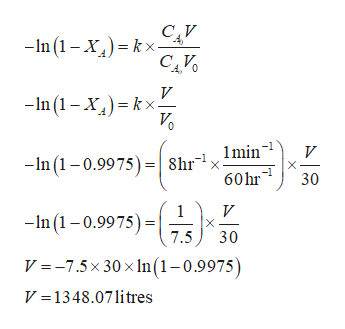Environmental Engineering:Cyanide is used in the production of intermediates in the pharmaceutical industry. A small resulting waste stream containing an organic cyanide, R-CN, is to be treated by high a temperature degradation process that has a constant rate of 8 hr-1 (at 170oC). What size of Plug Flow Reactor (PFR) is required to reduce the R-CN concentration from 2000mg/L to 5mg/L, for a waste stream with a flow rate of 30L/min? Assume temperature remains constant in the PFR.

Question

Environmental Engineering:

Cyanide is used in the production of intermediates in the pharmaceutical industry. A small resulting waste stream containing an organic cyanide, R-CN, is to be treated by high a temperature degradation process that has a constant rate of 8 hr-1 (at 170oC). What size of Plug Flow Reactor (PFR) is required to reduce the R-CN concentration from 2000mg/L to 5mg/L, for a waste stream with a flow rate of 30L/min? Assume temperature remains constant in the PFR.

Step 1

Given:

The constant rate (k) at 170ºC is 8 hr-1.

Consider the volume of the plug flow reactor is denoted by V.

The initial concentration (CA0) is 2000 mg/l.

The final concentration (CA) is 5mg/l.

The flow rate (V0) of the waste stream is 30 L/min.

The rate of change of concentration is denoted by XA.

Calculate the rate of change of concentration XA as follows:

Step 2

Calculate the size of the plug ...help_outlineImage TranscriptioncloseCAV -In 1 -Xkx V n (1 Xkx 0 -In(10.99758hr1xlmin' 60hr V 30 V X 7.5 1 -n (10.9975) 30 V =-7.5x 30 x ln(1-0.9975) V 1348.07litres fullscreen

Want to see the full answer?

See Solution

Want to see this answer and more?

Our solutions are written by experts, many with advanced degrees, and available 24/7

See Solution
Tagged in

Civil Engineering